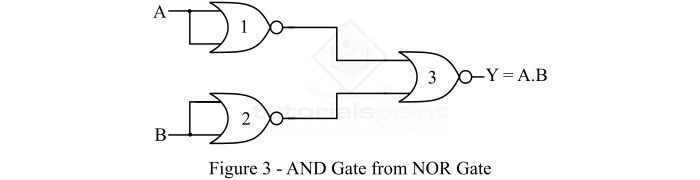# Implementation of AND Gate from NOR Gate

An AND Gate is a basic logic gate that performs the binary multiplication, i.e., it gives a HIGH or Logic 1 output, only when all its inputs are in HIGH or Logic 1 state. On the other hand, a NOR gate is a type of universal logic gate. Therefore, NOR gate can be used to realize any other type of logic gate.

Before going into the implementation of AND gate using NOR gates only, let us discuss the basic theory of AND Gate and NOR Gate first.

## What is an AND Gate?

An AND Gate is a basic logic gate. An AND gate may have two or more than two inputs, but gives only one output. The AND gate gives a LOW (Logic 0) output if any one of its inputs is in the LOW or Logic 0 state, otherwise, it gives a HIGH (Logic 1) state as output. Therefore, the output of the AND gate is HIGH or Logic 1 state, only if all its inputs are HIGH or Logic 1 state.

The AND gate is also known as an "all or nothing gate". The logic symbol of a two input AND gate is shown in Figure-1.If variables A and B are inputs to the AND gate and Y is the output variable of the AND gate, then the output equation of the AND gate is given by,

$$\mathrm{Y = A.B}$$

Where, the '.' (dot) symbol represents the AND operation. It is read as "Y is equal to A AND B".

The table that show the relationship between inputs and output of the AND gate is referred to as the truth table of AND gate. The following is the truth table of the AND Gate −

Input

Output

A

B

Y = A . B

0

0

0

0

1

0

1

0

0

1

1

1

## What is a NOR Gate?

NOR Gate is a type of universal logic gate, because this logic gate can be used for realization of any other type of logic gate.

NOR means NOT + OR. That means, the OR output is NOTed or inverted. Therefore, the NOR gate is a combination of OR gate and a NOT gate, i.e.,

$$\mathrm{NOR \:Gate = OR\:Gate + NOT\:Gate}$$

A NOR gate is a type of logic gate whose output is HIGH (Logic 1), only when all its inputs are LOW (Logic 0), and it gives an output LOW (Logic 0), even if any of its inputs become HIGH (Logic 1). The logic symbol of a two input NOR gate is shown in Figure-2.If variables A and B are the input variables and Y is the output variable of the NOR gate, then the output of the NOR gate is given by,

$$\mathrm{Y = \overline{A + B} = (A + B)'}$$

It is read as "Y is equal to A plus B whole bar".

The following is the truth table of the NOR gate −

Input

Output

A

B

Y = A . B

0

0

1

0

1

0

1

0

0

1

1

0

Now, let us discuss the implementation of AND Gate from NOR Gate.

## Implementation of AND Gate from NOR Gate

As mentioned above that the NOR gate is a logic gate, therefore, we may use it to realize an AND gate. The realization of an AND gate from the NOR gates only is shown Figure-3.Thus, for the realization of an AND gate from NOR gate, we require 3 NOR gates. Where, the first two NOR gates are used to complement the input variables A and B, and the third NOR gate is used to produce an output equivalent to the AND operation.

The outputs of the first two NOR gates are,

$$\mathrm{Y_1 = \bar{A}}$$

$$\mathrm{Y_2 = \bar{B}}$$

The output of the third NOR gate is,

$$\mathrm{Y = \overline{\bar{A} + \bar{B}} = \bar{\bar{A}} \cdot \bar{\bar{B}} = A \cdot B}$$

This is the output of an AND gate. Hence, the logic circuit using NOR gates shown in Figure-3 is equivalent to the AND gate.

Updated on: 02-Feb-2023

8K+ Views